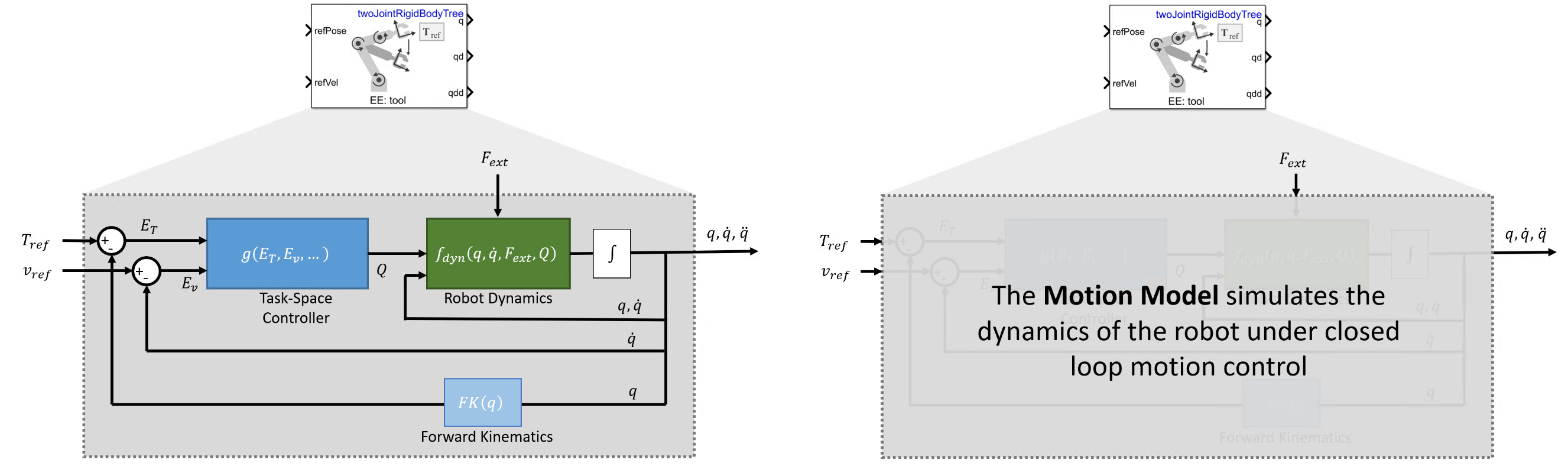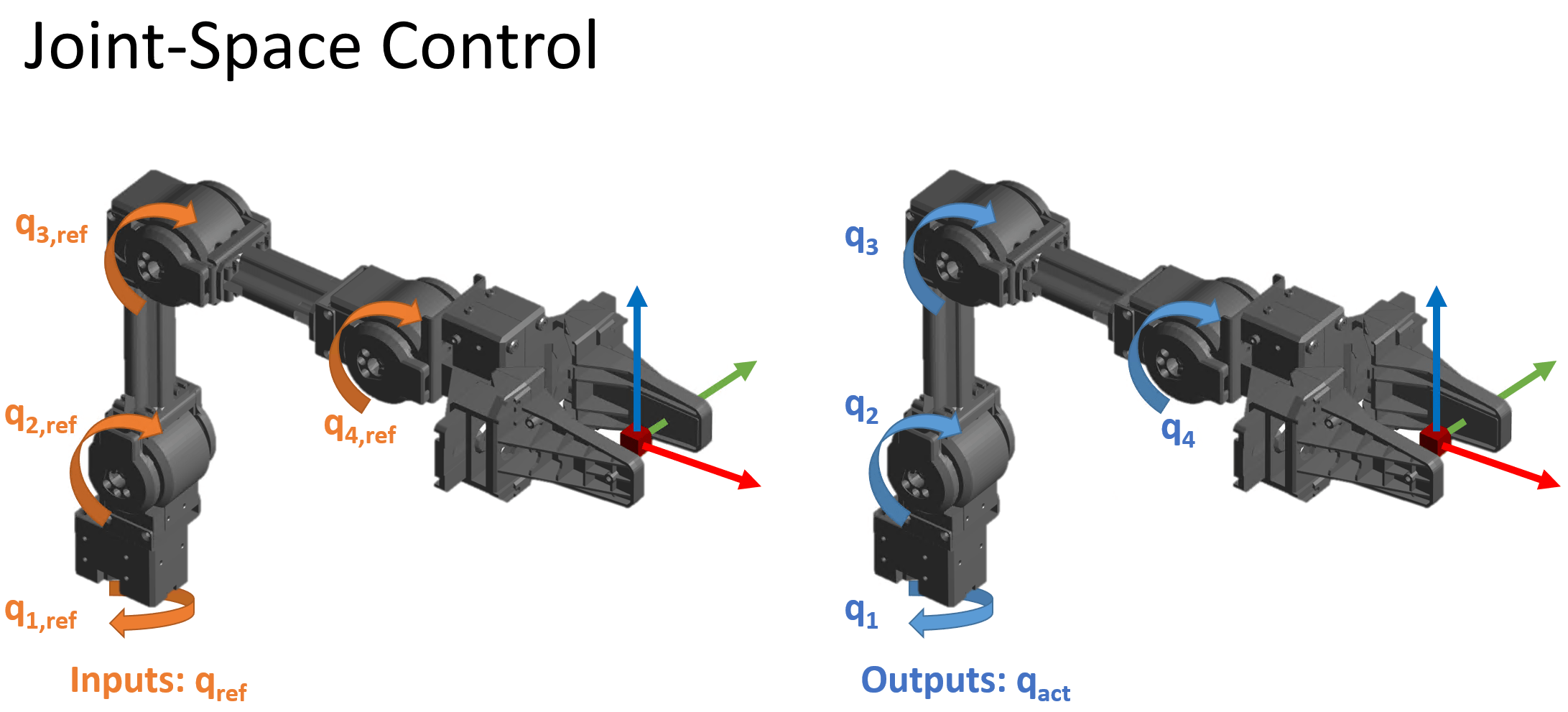The task-space motion model characterizes the closed-loop motion of a manipulator under task-space control, where the control action is defined in the SE(3) space with respect to the pose of a specified end effector. This topic covers the variables and equations for computing the behavior of joint position, velocity, and acceleration given motion modeled in the Plan and Execute Task- and Joint-Space Trajectories Using KINOVA Gen3 Manipulator object. For joint-space motion models, see the `jointSpaceMotionModel` object.

For an example that covers the difference between task-space and joint-space control, see Plan and Execute Task- and Joint-Space Trajectories Using KINOVA Gen3 Manipulator.### Key Variables

The model state consists of these values:

• $q$ — Robot joint configuration, as a vector of joint positions. Specified in $rad$ for revolute joints and $m$ for prismatic joints.

• $\underset{}{\overset{˙}{q}}$ — Vector of joint velocities in $rad\cdot {s}^{-1}$ for revolute joints and $m\cdot {s}^{-1}$ for prismatic joints

• $\underset{}{\overset{¨}{q}}$ — Vector of joint accelerations in $rad\cdot {s}^{-2}$ for revolute joints or $m\cdot {s}^{-2}$ for prismatic joints

The end-effector pose $T\left(q\right)$ of the robot is a 4-by-4 homogeneous matrix defined relative to the origin at the robot base. Positions are in meters. Two forms of $T$ are used for calculating errors in control:

• ${T}_{ref}$ — Reference end-effector pose, specified as a desired end-effector pose

• ${T}_{act}$ — Actual end-effector pose achieved by the motion

The end-effector transform decomposes as:

`$T=\left[\begin{array}{cc}R& X\\ 0& 1\end{array}\right]$`

where $R$ is the orientation as a 3-by-3 rotation matrix, and $X$ is a 3-by-1 vector of xyz-positions in meters.

The task-space velocity $v$ and acceleration $a$ consist of two 6-by-1 vectors:

$v=\left[\begin{array}{c}\omega \\ \underset{}{\overset{˙}{X}}\end{array}\right]$, $a=\left[\begin{array}{c}\alpha \\ \underset{}{\overset{¨}{X}}\end{array}\right]$

where $\omega$ and $\alpha$ are 3-by-1 vectors of angular velocities and accelerations of the frame, respectively.

### Equations of Motion

Use the task-space motion model to represent robots that are subject to a control law that acts on the task-space error. For example, when the input to the control law specifies end-effector motion. While there are many ways to implement such a system, in this model, the closed-loop response is approximated in the Plan and Execute Task- and Joint-Space Trajectories Using KINOVA Gen3 Manipulator object by providing a system under proportional-derivative Jacobian-Transpose style control. Regardless of how the system you are using works, this model can be used as a low-fidelity approximation of a system under closed-loop task-space control.

#### Proportional-Derivative Control

When the motion model uses proportional-derivative (PD) control, as determined by the MotionType property of the `taskSpaceMotionModel` object, the model computes forward dynamics using standard rigid body dynamics, but with subject to a PD control law that acts on the error between desired and actual end-effector pose.

• Inputs — This model accepts reference pose ${T}_{ref}$ and reference end-effector velocities ${v}_{ref}$

• Outputs — The model returns the $q,\underset{}{\overset{˙}{q}},\underset{}{\overset{¨}{q}}$ as the joint configuration, velocities, and accelerations as vectors

• Complexity — This is a medium complexity motion model. It uses complete rigid body dynamics, but the control law used in the model is relatively simple.In this system, the joint positions, velocities, and accelerations are computed using standard rigid-body Robot Dynamics. The generalized force input $Q$ is given by the PD Control law on the task-space error, scaled to the joint-space via a Jacobian-Transpose style control:

`$\frac{d}{dt}\left[\begin{array}{c}q\\ \underset{}{\overset{˙}{q}}\end{array}\right]={f}_{dyn}\left(q,\underset{}{\overset{˙}{q}},Q,{F}_{ext}\right)$`

`$Q=J\left(q{\right)}^{T}\left({K}_{P}{E}_{T}+{K}_{D}{E}_{v}\right)-B\underset{}{\overset{˙}{q}}+G\left(q\right)$`

`${E}_{T}=\left[\begin{array}{c}{e}_{rot}\\ {e}_{trans}\end{array}\right]$`

`${E}_{v}=\left({v}_{ref}-J\left(q\right)\underset{}{\overset{˙}{q}}\right)$`

where:

• ${e}_{rot}$ — is the rotational error converted to Euler angles using `rotm2eul(`${R}_{ref}{R}_{act}^{T}$)

• ${e}_{pos}$ — is the error in xyz-coordinates, calculated as ${X}_{ref}-{X}_{act}$.

• $G\left(q\right)$ — are the gravity torques and forces for all joints to maintain their positions in the specified gravity. For more information, see the `gravityTorque` function.

• $J\left(q\right)$ — is the geometric Jacobian for the given joint configuration for more information, see the `geometricJacobian` function.

The control input relies on these user-defined parameters:

• ${K}_{P}$ — Proportional gain, specified as a 6-by-6 matrix

• ${K}_{D}$ — Derivative gain, specified as a 6-by-6 matrix

• $B$ — Joint damping vector, specified as a two-element vector of damping constants in $N\cdot s\cdot ra{d}^{-1}$ for revolute joints and $N\cdot s\cdot {m}^{-1}$ for prismatic joints

You can specify these parameters as properties on the Plan and Execute Task- and Joint-Space Trajectories Using KINOVA Gen3 Manipulator object.

This model accepts the following inputs:

• ${T}_{ref}$ — Reference end-effector pose, specified as the desired end-effector pose

• ${v}_{ref}$ — Reference end-effector velocities, specified as a vector $v=\left[\begin{array}{c}\omega \\ \underset{}{\overset{˙}{X}}\end{array}\right]$, with angular velocities $\omega$ and translational velocities $\underset{}{\overset{˙}{X}}$